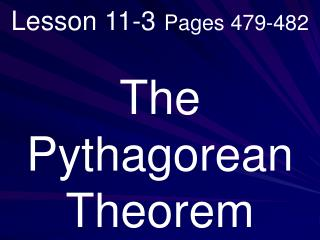# Lesson 11-3 Pages 479-482 - PowerPoint PPT PresentationDownload PresentationLesson 11-3 Pages 479-482

Lesson 11-3 Pages 479-482Download Presentation## Lesson 11-3 Pages 479-482

- - - - - - - - - - - - - - - - - - - - - - - - - - - E N D - - - - - - - - - - - - - - - - - - - - - - - - - - -
##### Presentation Transcript

1. Lesson 11-3Pages 479-482 The Pythagorean Theorem

2. What you will learn! How to find length using the Pythagorean Theorem.

3. Vocabulary

4. What you really need to know! The sides of a right triangle have special names. The sides adjacent to the right angle are the legs. The side opposite the right angle is the hypotenuse.

5. The Pythagorean Theorem describes the relationship between the length of the hypotenuse and the lengths of the legs. In a right triangle, the square of the length of the hypotenuse equals the sum of the squares of the lengths of the legs.

6. The Pythagorean Theorem c a b c2 = a2 + b2

8. Example 1: A gymnastics tumbling floor is in the shape of a square with sides 12 meters long. If a gymnast flips from one corner to the opposite corner, about how far has he flipped?

9. c2 = a2 + b2 c2 = 122 + 122 c2 = 144+ 144 c2 = 288 c≈ 17m

10. Example 2: Find the missing measure of the triangle. 15 cm a 9 cm

11. 152 = a2 + 92 225= a2 + 81 144= a2 12= a a=12 cm

12. Example 3: Televisions are measured by their diagonal measure. If the diagonal of a television is 36 inches, and its height is 21.6 inches, what is its width?

13. c2 = a2 + b2 362 = 21.62 + b2

14. 362 = 21.62 + b2 1,296 = 466.56 + b2 829.44 = b2 28.8 = b 28.8 inches

15. Example 4: Determine whether the triangle is a right triangle. 2.5 cm, 6 cm, 6.5 cm

16. c2 = a2 + b2 6.52 = 62 + 2.52 42.25 = 36 + 6.25 42.25 = 42.25 YES! Right Triangle.

17. Example 5: Determine whether the triangle is a right triangle. 5 ft, 6 ft, 8 ft

18. c2 = a2 + b2 82 = 62 + 52 64 = 36 + 25 64 ≠ 61 No! Not Right Triangle.

19. Page 481 Guided Practice #’s 4-8

20. Read: Pages 479-481 with someone at home and study examples!

21. Homework: Page 482 #’s 9-21 all #’s 24-34 all Lesson Check 11-3

22. Link to Math Review Practice

23. Page 590 Lesson 11-3